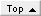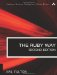# Regular ExpressionsRuby Way By Hal Fulton Slots : 1.0 Table of Contents

I would choose

To lead him in a maze along the patterned paths…

Amy Lowell, "Patterns"

The power of regular expressions as a computing tool has often been underestimated. From their earliest theoretical beginnings in the 1940s, they found their way onto computer systems in the 1960s and thence into various tools in the UNIX operating system. In the 1990s, the popularity of Perl helped make regular expressions a household item rather than the esoteric domain of bearded gurus.

The beauty of regular expressions is that everything in our experience can be understood in terms of patterns. Where there are patterns that we can describe, we can detect matches; we can find the bits of reality that correspond to those matches; and we can replace those bits with others of our own choosing.

#### Escaping Special Characters

The class method Regexp.escape will escape any special characters that are used in regular expressions. Such characters include the asterisk, question mark, and brackets.

` `

` str1 = "[*?]" str2 = Regexp.escape(str1)  # "\[\*\?\]" `

The method Regexp.quote is an alias.

#### Compiling Regular Expressions

Regular expressions can be compiled using the class method Regexp.compile, which is really only a synonym for Regexp.new. The first parameter is required and can be a string or a regex. (Note that if the parameter is a regex with options, the options won't carry over into the newly compiled regex.)

` `

` pat1 = Regexp.compile("^foo.*")  # /^foo.*/ pat2 = Regexp.compile(/bar\$/i)   # /bar/ (i not propagated) `

The second parameter, if present, is normally a bitwise OR of any of the following constants: Regexp::EXTENDED, Regexp::IGNORECASE, and Regexp::MULTILINE. Additionally, any non-nil value will have the result of making the regex not case sensitive; we don't recommend this practice.

` `

` options = Regexp::MULTILINE || Regexp::IGNORECASE pat3 = Regexp.compile("^foo", options) pat4 = Regexp.compile(/bar/, Regexp::IGNORECASE) `

The third parameter, if specified, is the language parameter, which enables multibyte character support. It can take any of the following four string values:

` `

`  "N" or "n" means None  "E" or "e" means EUC  "S" or "s" means Shift-JIS  "U" or "u" means UTF-8 `

#### Accessing Backreferences

The class method Regexp.last_match will return an object of class MatchData (as will the instance method match). This object has instance methods that enable the programmer to access backreferences.

The MatchData object is manipulated with a bracket notation as though it were an array of matches. The special element 0 contains the text of the entire matched string. Thereafter, element n refers to the nth match.

` `

` pat = /(.+[aiu])(.+[aiu])(.+[aiu])(.+[aiu])/i # Four identical groups in this pattern refs = pat.match("Fujiyama") # refs is now: ["Fujiyama","Fu","ji","ya","ma"] x = refs y = refs[2..3] refs.to_a.each { |x| print "#{ x} \n"} `

Note that the object refs isn't a true array. Thus, when we want to treat it as one by using the iterator each, we must use to_a (as shown previously) to convert it to an array.

We can use more than one technique to locate a matched substring within the original string. The methods begin and end will return the beginning and ending offsets of a match. (It is important to realize that the ending offset is really the index of the next character after the match.)

` `

` str = "alpha beta gamma delta epsilon" #      0....5....0....5....0....5.... #      (for  your counting convenience) pat = /(b[^ ]+ )(g[^ ]+ )(d[^ ]+ )/ # Three words, each one a single match refs = pat.match(str) # "beta " p1 = refs.begin(1)         # 6 p2 = refs.end(1)           # 11 # "gamma " p3 = refs.begin(2)         # 11 p4 = refs.end(2)           # 17 # "delta " p5 = refs.begin(3)         # 17 p6 = refs.end(3)           # 23 # "beta gamma delta" p7 = refs.begin(0)         # 6 p8 = refs.end(0)           # 23 `

Similarly, the offset method will return an array of two numbers, which are the beginning and ending offsets of that match. To continue the preceding example:

` `

` range0 = refs.offset(0)    # [6,23] range1 = refs.offset(1)    # [6,11] range2 = refs.offset(2)    # [11,17] range3 = refs.offset(3)    # [17,23] `

The portions of the string before and after the matched substring can be retrieved by the instance methods pre_match and post_match, respectively. To continue the preceding example:

` `

` before = refs.pre_match    # "alpha " after  = refs.post_match   # "epsilon" `

#### Using Character Classes

Ruby regular expression might contain references to character classes, which are basically named patterns (of the form [[:name:]]). For example, [[:digit:]] means the same as [0-9] in a pattern. In most cases, this turns out to be shorthand.

Some others are [[:print:]] (printable characters) and [[:alpha:]] (alphabetic characters).

` `

` s1 = "abc\007def" /[[:print:]]*/.match(s1) m1 = Regexp::last_match               # "abc" s2 = "1234def" /[[:digit:]]*/.match(s2) m2 = Regexp::last_match               # "1234" /[[:digit:]]+[[:alpha:]]/.match(s2) m3 = Regexp::last_match               # "1234d" `

#### Treating Newline as a Character

Ordinarily a dot will match any character except a newline. When the m (multiline) modifier is used, a newline will be matched by a dot. The same is true when the Regexp::MULTILINE option is used in creating a regex.

` `

` str = "Rubies are red\nAnd violets are blue.\n" pat1 = /red./ pat2 = /red./m str =~ pat1       # false str =~ pat2       # true `

Suppose that we want to determine whether a string is a valid IPv4 address. The standard form of such an address is a dotted quad or dotted decimal string. This is, in other words, four decimal numbers separated by periods, each number ranging from 0 to 255.

The pattern given here will do the trick (with a few exceptions such as 127.1). We break the pattern up a little just for readability. Note that the \d symbol is double escaped so that the slash in the string will get passed on to the regex.

` `

` num = "(\\d|?\ \ d\ \ d|2[0-4]\\d|25[0-5])" pat = "^#{ num} \.#{ num} \.#{ num} \.#{ num} \$" ip_pat = Regexp.new(pat) ip1 = "9.53.97.102" if ip1 =~ ip_pat                # Prints "yes"   puts "yes" else   puts "no" end `

IPv6 addresses aren't in widespread use yet, but we include them for completeness. These consist of eight colon-separated 16-bit hex numbers with zeroes suppressed.

` `

` num = "[0-9A-Fa-f]{ 0,4} " pat = "^" + "#{ num} :"*7 + "#{ num} \$" ipv6_pat = Regexp.new(pat) v6ip = "abcd::1324:ea54::dead::beef" if v6ip =~ ipv6_pat             # Prints "yes"   puts "yes" else   puts "no" end `

#### Matching a Keyword-Value Pair

Occasionally, we want to work with strings of the form "attribute=value" (for example, when we parse some kind of configuration file for an application).

This code fragment will extract the keyword and the value. The assumptions are that the keyword or attribute is a single word; the value extends to the end of the line; and the equal sign can be surrounded by whitespace.

` `

` pat = /(\w+)\s*=\s*(.*?)\$/ str = "color = blue" matches = pat.match(str) puts matches            # "color" puts matches            # "blue" `

For additional information see the section "Adding a Keyword-Value String to a Hash."

#### Matching Roman Numerals

Here we match against a complex pattern to determine whether a string is a valid Roman number (up to decimal 3999). As before, the pattern is broken up into parts for readability.

` `

` rom1 = "m{ 0,3} " rom2 = "(d?c{ 0,3} |c[dm])" rom3 = "(l?x{ 0,3} |x[lc])" rom4 = "(v?i{ 0,3} |i[vx])" rom_pat = "^#{ rom1} #{ rom2} #{ rom3} #{ rom4} \$" roman = Regexp.new(rom_pat, Regexp::IGNORECASE) year1985 = "MCMLXXXV" if year1985 =~ roman      # Prints "yes"   puts "yes" else   puts "no" end `

#### Matching Numeric Constants

A simple decimal integer is the easiest number to match. It has an optional sign and consists thereafter of digits (except that Ruby allows an underscore as a digit separator). Note that the first digit shouldn't be a zero; then it would be interpreted as an octal constant.

` `

` int_pat = /^[+-]?[1-9][\d_]*/ `

Integer constants in other bases are similar. Note that the hex and binary patterns are not case sensitive because they contain at least one letter.

` `

` hex_pat = /^[+-]?0x[\da-f_]+\$/i oct_pat = /^[+-]?0[0-7_]+\$/ bin_pat = /^[+-]?0b[01_]+/i `

A normal floating-point constant is a little tricky; the number sequences on each side of the decimal point are optional, but one or the other must be included.

` `

` float_pat = /^(\d[\d_]*)*\.[\d_]*\$/ `

Finally, scientific notation builds on the ordinary floating-point pattern.

` `

` sci_pat = /^(\d[\d_]*)?\.[\d_]*(e[+-]?)?(_*\d[\d_]*)\$/i `

These patterns can be useful if, for instance, you have a string and you want to verify its validity as a number before trying to convert it.

#### Matching a Date/Time String

Suppose that we want to match a date/time in the form mm/dd/yy hh:mm:ss. This pattern is a good first attempt: datetime_re=/(\d\d)\/(\d\d)\/(\d\d) (\d\d):(\d\d):(\d\d)/.

However, that will also match invalid date/times, and miss valid ones. A pickier pattern is shown in Listing 2.8.

##### Listing 2.8 Matching Date/Time Strings
` class String   def scan_datetime(flag=2)     datetime_re=/((\d\d)\/(\d\d)\/(\d\d) (\d\d):(\d\d):(\d\d))/     month_re=/(0?[1-9]|1[0-2])/     # 01 to 09 or 1 to 9 or 10-12     day_re=/([0-2]?[1-9]|[1-3])/     # 1-9 or 01-09 or 11-19 or 21-29 or 10,11,20,21,30,31      year_re=/(\d\d)/      # 00-99      hour_re=/(?[1-9]|[0-4])/      # 1-9 or 00-09 or 11-19 or 10-14 or 20-24      minute_re=/([0-5]\d)/      # 00-59, both digit required      second_re=/(:[0-6]\d)?/      # leap seconds ;-) both digits required if present      date_re=/(#{ month_re.source} \/#{ day_re.source} \    /#{ year_re.source} )/      time_re=/(#{ hour_re.source}    :#{ minute_re.source} #{ second_re.source} )/      datetime_re2 = /(#{ date_re.source}  #{ time_re.source} )/      if flag==2        self.scan(datetime_re2)    # returns arrays      else        self.scan(datetime_re)      end    end end str="Recorded on 11/18/00 20:31:00, viewed 11/18/00 8:31 PM " str.scan_datetime # [ ["11/18/00 20:31:00", "11", "18", "00", "20", "31", "00"] ] str.scan_datetime(2) # [ ["11/18/00 20:31:00", "11/18/00", "11", "18", "00", #  "20:31:00", "20", "31", ":00"], # ["11/18/00 8:31", "11/18/00", "11", "18", "00", "8:31", #  "8", "31", nil]  ] `

#### Detecting Doubled Words in Text

Here, we implement the famous double-word detector. Typing the same word twice in succession is one of the most common typing errors. The code we show here will detect instances of that occurrence.

` `

` double_re = /\b(['A-Z]+) +\1\b/i str="There's there's the the pattern." str.scan(double_re)  #  [["There's"],["the"]] `

Note that the trailing i in the regex is for not case sensitive matching. There is an array for each grouping, hence the resulting array of arrays.

#### Matching All-caps Words

This one is simple if we assume no numerics, underscores, and so on.

` `

` allcaps = /\b[A-Z]+\b/ string = "This is ALL CAPS" string[allcaps]                #  "ALL" `

Suppose that you want to simply extract every word in all-caps.

` `

` string.scan(allcaps)   #  ["ALL", "CAPS"] `

If we wanted, we could extend this concept to include Ruby identifiers and similar items.The Ruby Way, Second Edition: Solutions and Techniques in Ruby Programming (2nd Edition)
ISBN: 0672328844
EAN: 2147483647
Year: 2000
Pages: 119
Authors: Hal Fulton

Similar book on Amazon